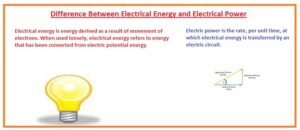Hello guys, I hope you all are doing great. In today’s tutorial, we will have a look at Difference Between Electrical Energy and Electrical Power. In the electrical power system, there are 2 most common terms used first one is electrical energy and the second one is electrical power. The basic difference among them is that electrical energy defines as the amount of work done due to current flows through the circuitry.

While electrical power explains about the rate over which work is done in the circuitry. In today’s post, we will have a detailed look at both electrical energy and electrical power and find their differences. So let’s get started with Difference Between Electrical Energy and Electrical Power.

#### Difference Between Electrical Energy and Electrical Power

Electrical Energy

•  The net work done in circuitry is called electrical energy.
•  It denoted with the E.
• It caused due to the motion of electrons.
• It is converted form oef electrical poteteial enrgy.
• It is a combination of current and electric potential.
• Any type of electrical energy is potential energy.
• It measured in kilowatt-hour.
• 1 kWh is equal to 3.6 Mega jouls.
• The energy meter is used to measure this term
• the quantity of electric energy produced depend on the charge motion
• Lightning is an example of electrical energy  car battery produces electric energy

• Its mathematical expression is E = VI.t or Pxt.
• Its system international unit is the joule.Electrical power

• The work done per unit time to flow of current in circuitry is called electrical power.
• Rate of energy transfer in unit time called electric power.
• P=VQ/t
• It is generated through electric generators, like an induction generator,, synchronous generator.
• It can also get from the battery.
• IT used at home markets industries to run loads.
• It can be transmitted to a longer distance through transmission lines.
• The main types of power are ac power dc power apparent reactive power
• It denoted with the P.
• Its mathematical expression is P=VxI.
• Its system international unit is a watt.

• Charges move from high level to low level then current passes from the positive end to the negative end.
• The P.E due to charges among the points of device transformed to kinetic energy. Such devices called passive loads

1.James Franklin says: